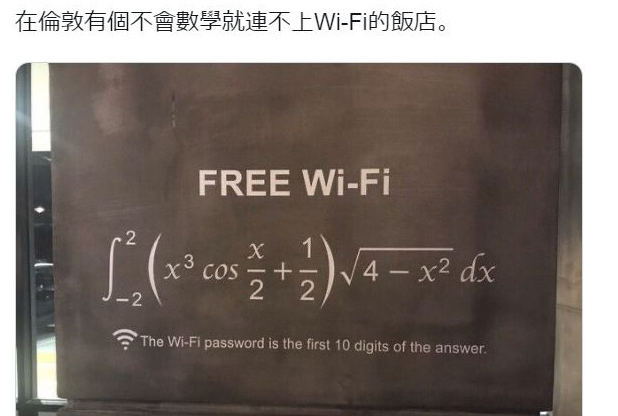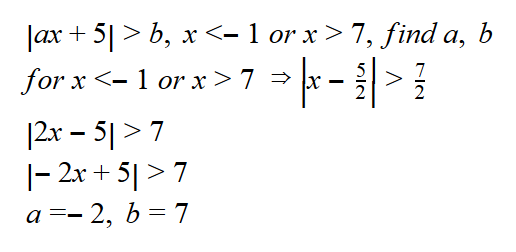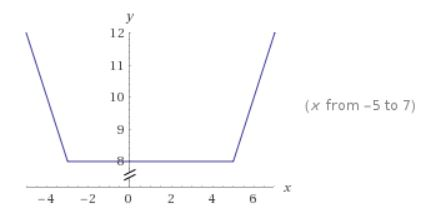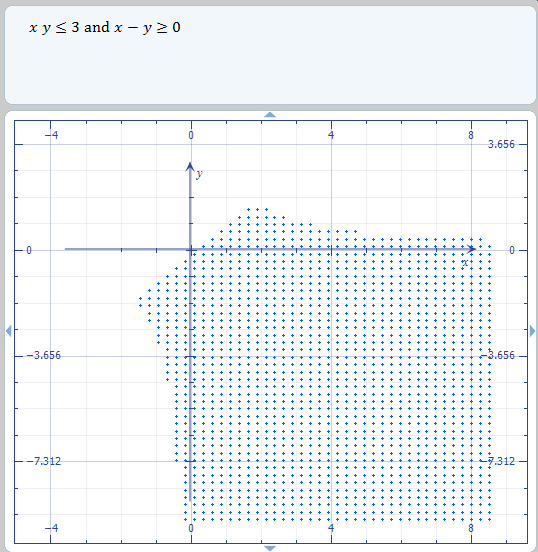好用線上數學編輯器 – Mathcha.io

https://www.mathcha.io/

https://www.mathcha.io/documentation/

https://www.mathcha.io/editor/3QYd6c5ds7xUwgpP27s2VG1V4T57m25vCO0JjD2https://diary.tw/s/intwifi

[數學]絕對值特性階乘從1乘到99999的位數

p = 1
for i in range(1,100000):
p *= i
print(p)
print(len(str(p)))

[2018/5/18 12:12]

import math
p = math.factorial(99999)
print(p)
print(len(str(p)))
type(p)

數學題-簡單絕對值不等式

http://www.wolframalpha.com/input/?i=y%3Dabs(x%2B3)%2Babs(x-5)[數學]證明A(a), B(b)的中點座標是(a+b)/2

abs((a+b)/2 – a) = abs((b-a)/2)

abs((a+b)/2 – b) = abs((a-b)/2)

abs((b-a)/2) = abs((a-b)/2) 所以距離相等, 故 C點為 AB的中點, 得證.

微軟工具Microsoft Mathematics

1. Mathway: http://diary.tw/archives/720
2. Wolframalpha: http://diary.tw/archives/1196

x * y <=3
x – y >=0

http://www.wolframalpha.com/input/?i=x*y%3C%3D3%2C+x-y%3E%3D0